[ Home ]  [ Slide Rule Annex]

# Trig Scales

Trig scales were in use almost as early as the logarithmic scales. Trig scales went through various inventions and improvements. Let us review the progress.

 Mannheim Rules Early slide rules were so called "Mannheim" type, which had A =B, C= D scales on front side and =S(sin), L(log), T(tan)= on rear of the slide. S scale is referenced to A/B scales and graduated in degree/ minutes. Since A scale has two cycles, sin covers a wide range of 35 minutes to 90 degrees. T scale is referenced at C/D scale and covers 6 degrees to 45 degrees.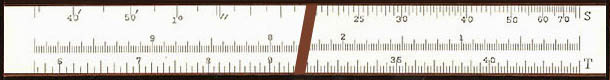Exhibit 1. Trig sacles for K&E 4053-3 Why this asymmetry? One explanation is that, when the slide is reversed so that trig scales are on the front side, S and T scales are opposit A and D scales, respectively. This enables direct reading of trig values on A or D scales. Besides, scales used for calculations were mainly A and B scals in early days. A/B scales were prefered bacause "over scales" happen less frequently.

 Deci-Trig (Decimal Trig) When angles are subject of calculation, use of decimal farction of degrees is necessary. Thus scales with decimal degree graduation. K&E called it "Deci-Trig". The decimal degree scales are diversification of the scales, rather than improvement on them. K&H continued to supply both sexagesimal and decimal rules.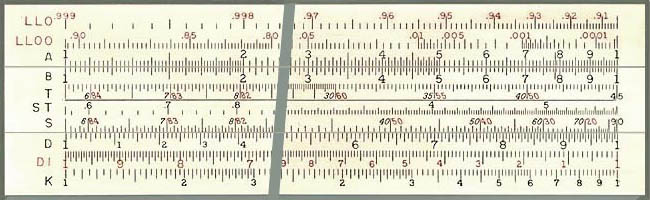Exhibit 2. K&E N4081-3 Unlike Mannheim rules, all trig scales are referenced to C/D scales. One feature of this particular rule is that cotangent and cosine degrees share the T and S scales and the red figures are slanted to the left, signifying increase of value is to the left.

 SRT Scales For later models of Keuffel & Esser rules, ST scale for small angles is renamed as SRT.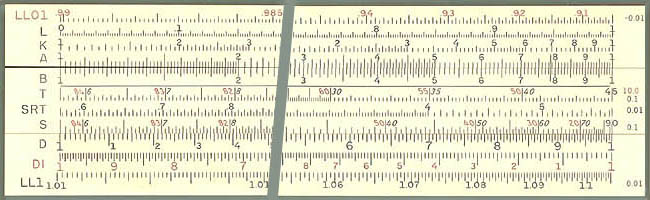Exhibit 3. K&E 4081-3 "R" in SRT means Radian and it can be used for convertion between degrees and radians for small angles. To do this, align the slider with the stator. Degree angle is on SRT and radian is on D scale.

 SI/TI Scales Hemmi had their own trig scales, SI and TI, inverted trig scales. In this scale angles are graduated from right to left. The inverted trig scales on the slider gives trig values on D scale aginst C scale index. This is quite a difference from the reguler trig scales which gives values on C scale against D scale index. Obvious advantage is that one can carry out chain calculations such as a*b*sin or a*sin/b without transposing numbers. Some may argue that for the regular trig scales one can carry out chain calculations such as a*b/sin or a/(sin*b). Well, this point may be a draw. (; - ) Hemmi claims that, because of this, many calculations can be carried out directly. Examples given in the mannual includes, sin proportion, solution of right triangle and others.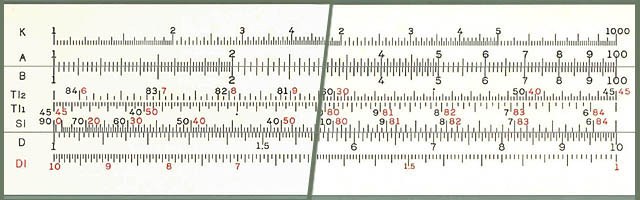Exhibit 4. Hemmi 250

 SI1, SI2/ TI1, TI2 Scales There is yet another variant to the Hemmi's SI/TI scales. It is on a Hemmi's Wat-time economy rule and the earlier model of 2664. The scales are designated as SI1, SI2, TI1 and TI2.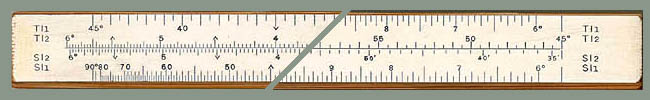Exhibit 5. Hemmi SI1, SI2, TI1, TI2 scales (Click above to view full range image. ) SI1 and TI1 are the regular inverted trig scales covering 6 degrees and up. SI2 and TI2 cover angles less than 6 degrees, very much the same way the ST scales do. But why two saparate scales? To get more accuracy on values near 6 degrees? May be. Sin and Tangent values at 5' 45" are about 0.5% apart. There may have been applications requiring better than that. And there is another neat idea built into TI2 scale. The right half of TI2 scale, just about beyond 2 degees, is replaced with the tan scale for between 45 and 70 degrees. So tangent smaller than 2 degrees share the SI2 scale and you get extra range up to 70 degrees for tangent.

[ Slude Tule Annex]  [ Top]